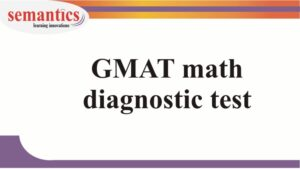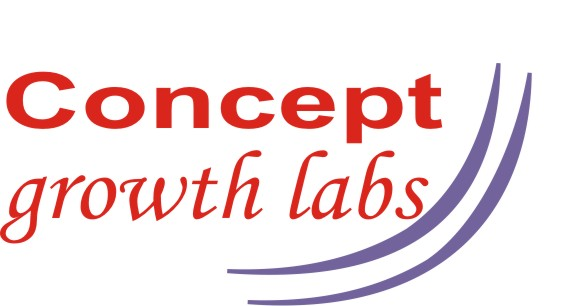# GMAT math diagnostic testTake this diagnostic test and let us know your scores.

We can have a counselling session based on this data.

Book your counselling session by filling this formMath Diagnostic test

Duration 15 mins

1 / 7

If 3x+2y = 7 and 2x – y = 7, what is the value of x?

2 / 7

What is the diameter of a circular region that has area 10(pi)?

3 / 7

A telephone call costs \$1.25 for the first minute and \$0.32 for each additional minute. What is the cost, in dollars, of a telephone call that lasts for x minutes, where x is an integer?

4 / 7

If x2 < x, then x must be

5 / 7

The sum of 25 percent of 36 and 75 percent of 56 equals

6 / 7

If x and y are two-digit integers such that x > 40 and y < 70, which of the following is closest to the maximum possible value of xy?

7 / 7

Raymond took several days to move a certain lawn. He moved (1/3)rd of the lawn the first day, (1/2) of the remaining un-moved portion the second day, and (3/4th) of the remaining un-moved portion the third day. What fraction of the lawn remained un-moved at the end of the third day?

0%

## What next?

Do a sentence correction diagnostic  test here

Do a critical reasoning diagnostic test here

Do a reading comprehension  diagnostic test here